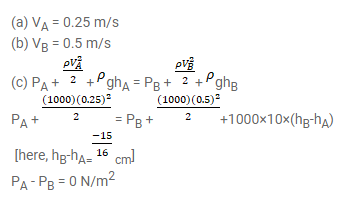# Supposed the tube in the previous problem is kept vertical with

Question:

Supposed the tube in the previous problem is kept vertical with A upward but the other conditions remain the same. The separation between the cross-section at $\mathrm{A}$ and $\mathrm{B}$ is $15 / 16 \mathrm{~cm}$. Repeat parts $(\mathrm{a})$, (b) and (c) of the previous problem. Take $\mathrm{g}=$ $10 \mathrm{~m} / \mathrm{s}^{2}$.

Solution: